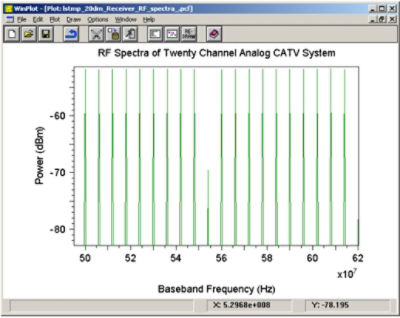# Analog CATV Examples

## Tool Used: OptSim

This example demonstrates using OptSim in block mode to analyze analog CATV transmission systems. Here we consider distortions such as composite second order, composite triple beat, and intermodulation distortion. In fiber-based analog CATV transmission systems, there is usually one wavelength used for the signal transmission with multiple analog RF channels modulated onto it. While BER is the most common performance metric in a digital transmission system, measurements of distortions are the critical metrics in an analog transmission system. These results can be produced through simulations in OptSim. Let us first consider a two tone direct modulated analog transmission system shown below: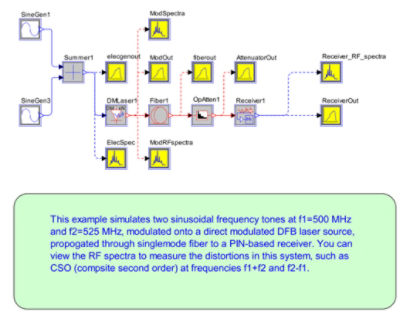In this example, there are two electrical sine-wave frequencies generated and summed. These two frequencies are at 500 MHz and 525 MHz. These are then modulated onto a direct modulated DFB laser at a wavelength of 1550 nm. This is then propagated over 40 km of singlemode optical fiber to a PIN-based optical receiver. The RF spectra can be viewed in the spectrum analyzer to measure the distortions such as composite second order (CSO) distortion, which are due to new frequencies generated at f1 + f2 and f2 - f1. Figure below shows the RF spectra with power at frequencies 1025 MHz and 25 MHz as well as the original frequencies of 500 MHz and 525 MHz. Also shown are the modulation distortions at 2*f1 and 2*f2 at 1000 MHz and 1050 MHz respectively.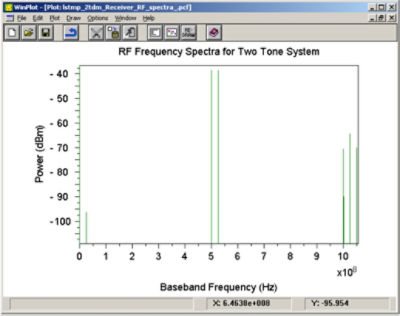Let us next consider a two tone externally modulated analog transmission system shown below: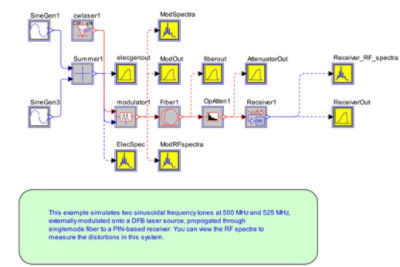The RF spectra can be viewed in the spectrum analyzer to measure the distortions such as composite second order (CSO) distortion, which are due to new frequencies generated at f1 + f2 and f2 - f1. Figure below shows the RF spectra with power at frequencies 1025 MHz and 25 MHz as well as the original frequencies of 500 MHz and 525 MHz. Also shown are the modulation distortions at 2*f1 and 2*f2 at 1000 MHz and 1050 MHz respectively. The most striking difference between this and the direct modulated example are the additional distortions at a number of additional frequencies.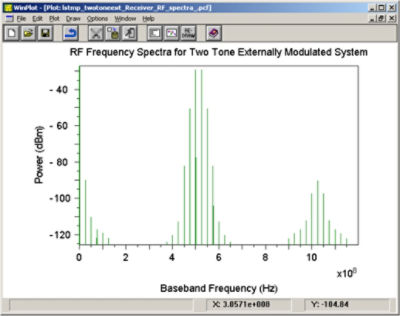Let us now consider the third case: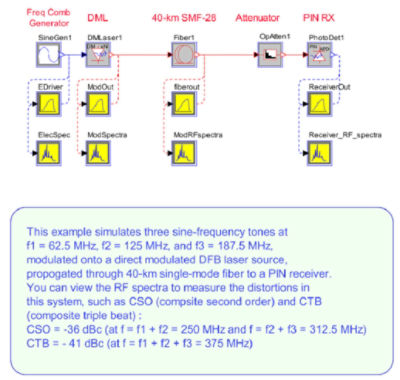Here, the three frequencies are then modulated together onto a DFB laser with wavelength 1550 nm and propagated over 40 km of standard singlemode fiber (SMF-28) to a PIN-based receiver. The RF spectrum at the receiver can be viewed to analyze the composite second order, composite triple beat, and other distortions in the system as shown below: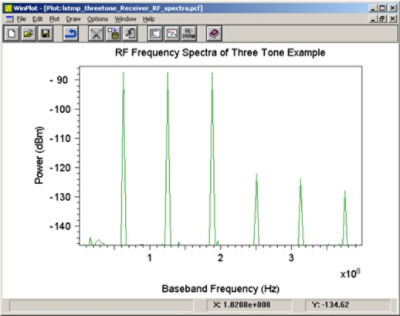Let us now consider the fourth case: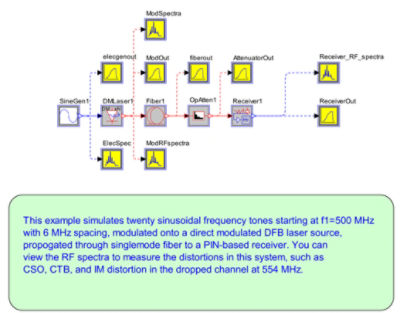This case demonstrates a simulation of a 20-channel analog CATV system. In this example as in the above, we focus our attention on the distortions. In the figure below, we see the modulated frequencies for the twenty channels minus the dropped channel, and the distortions present at that frequency.